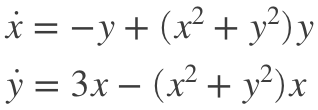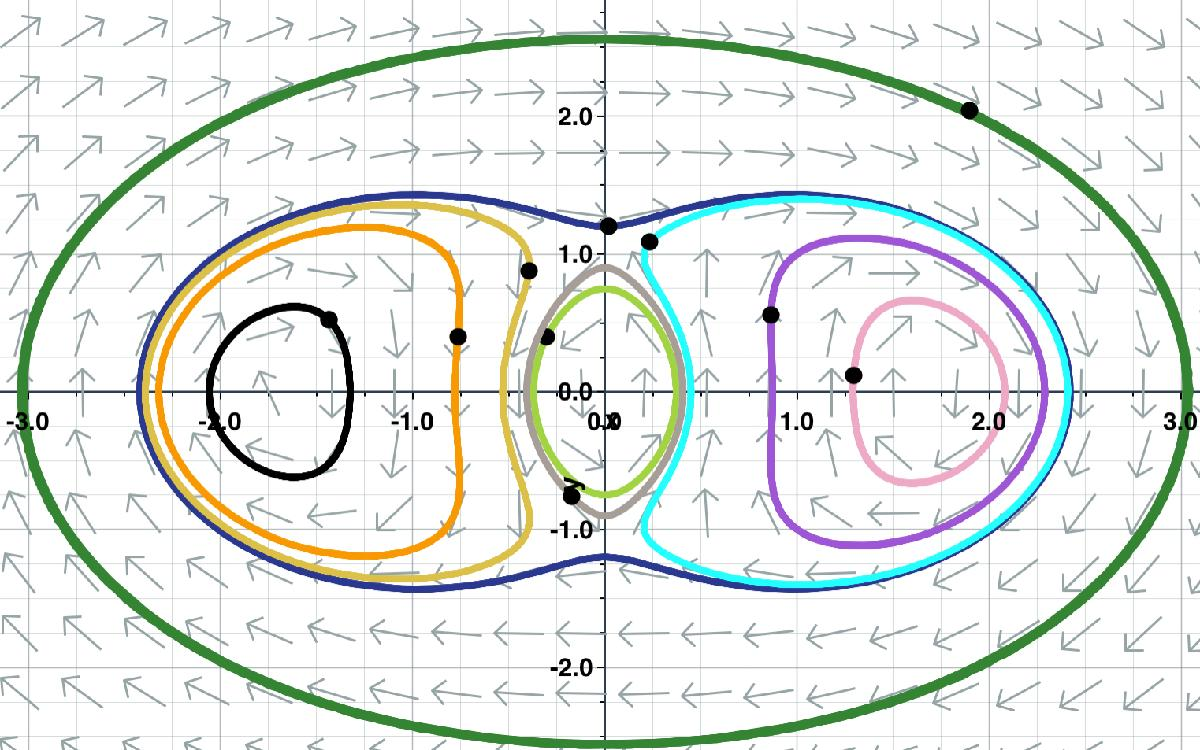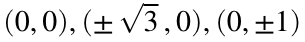# MATLAB ODE45 Problem 1

## Simulate the system

####The purpose of this assignment is not simply to show that you can get ode45 to run. The purpose is to demonstrate how the numerically solving an ODE is a useful companion to the qualitative methods we are learning from Strogatz when we're trying to understand the dynamics due to an ODE.
Here is what it looks like using the Slopes app.This has five fixed points.. The three fixed points on the x-axis are centers and the two fixed points on the y-axis are saddles.
This has a conserved energyE=@(x,y) -3/2*x.^2-1/2*y.^2+1/4*(x.^2+y.^2).^2;
fcontour(E,[-3 3 -1.5 1.5],'LevelStep',0.125)